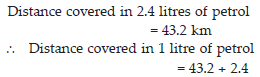Fraction and Decimal (Maths) Class 7 - NCERT Questions
Q 1.

Solve :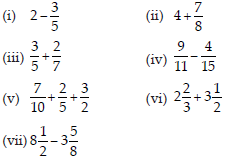SOLUTION: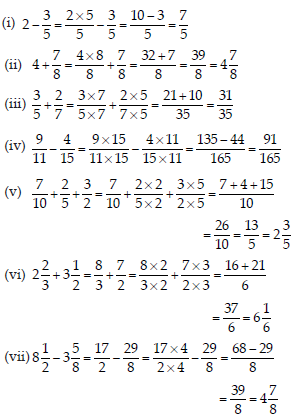Q 2.

Arrange the following in descending order:
(i) 2/9 , 2/3 , 8/21 (ii) 1/5 , 3/7 , 7/10

SOLUTION: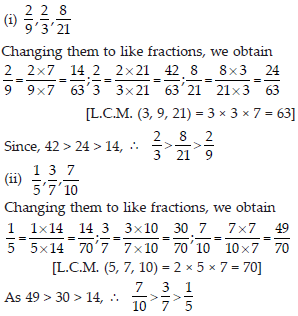Q 3.

In a ′′magic square′′, the sum of the numbers in each row, in each column and along the diagonals is the same. Is this a magic square?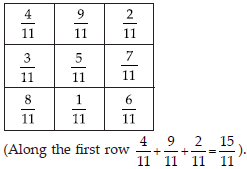SOLUTION: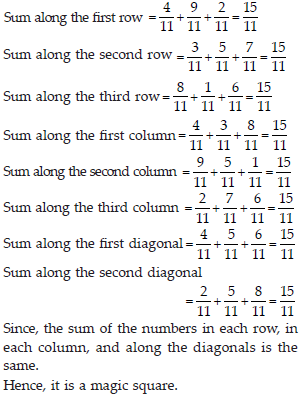Q 4.

A rectangular sheet of paper is 1212 cm long and 1023 cm wide. Find its perimeter.

SOLUTION: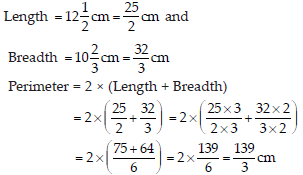Q 5.

Find the perimeters of (i) ∆ABE (ii) the rectangle BCDE in this figure. Whose perimeter is greater?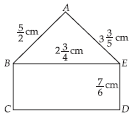SOLUTION: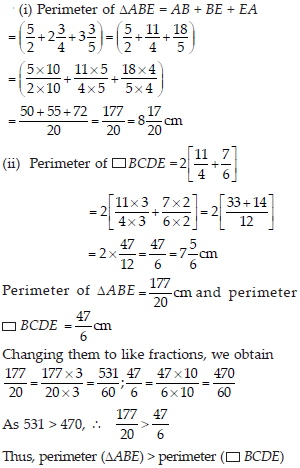Q 6.

Salil wants to put a picture in a frame. The picture is 735 cm wide. To fit in the frame the picture cannot be more than 7310 cm wide. How much should the picture be trimmed?

SOLUTION: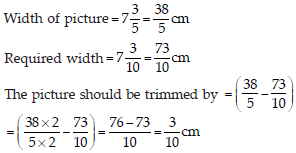Q 7.

Ritu ate 3/5 part of an apple and the remaining apple was eaten by her brother Somu. How much part of the apple did Somu eat? Who had the larger share? By how much?

SOLUTION: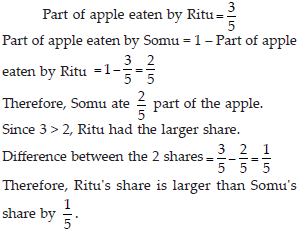Q 8.

Michael finished colouring a picture in 7/12 hour. Vaibhav finished colouring the same picture in 3/4 hour. Who worked longer? By what fraction was it longer?

SOLUTION: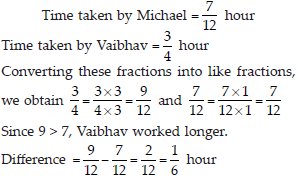Q 9.

Which of the drawings (A) to (D) show :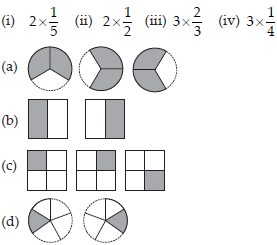SOLUTION: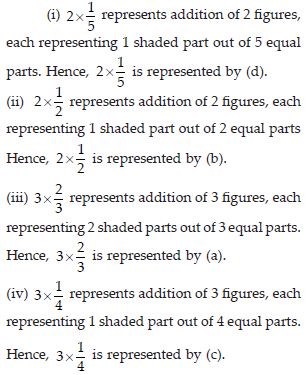Q 10.

Some pictures (A) to (C) are given below. Tell which of them show: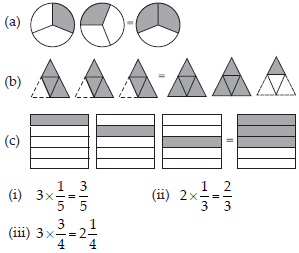SOLUTION: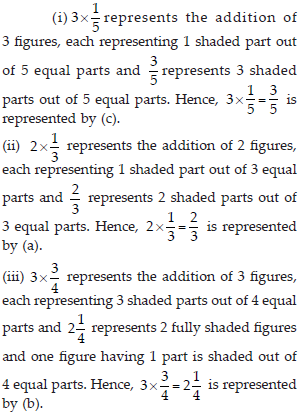Q 11.

Multiply and reduce to lowest form and convert into a mixed fraction: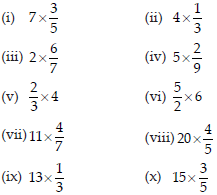SOLUTION: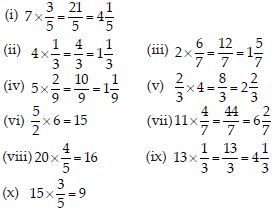Q 12.

Shade: (i) 1/2 of the circles in box (A); (ii) 2/3 of the triangles in box (B); (iii) 3/5 of the squares in box (C).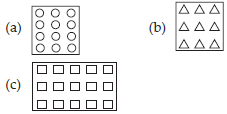SOLUTION: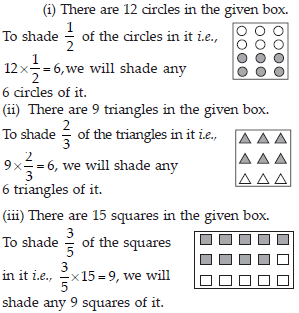Q 13.

Find: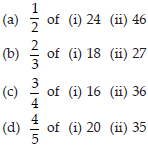SOLUTION: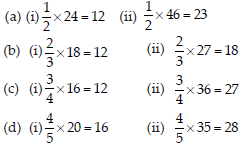Q 14.

Multiply and express as a mixed fraction :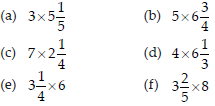SOLUTION: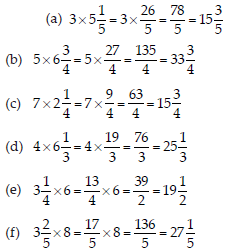Q 15.

Find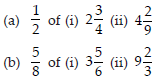SOLUTION: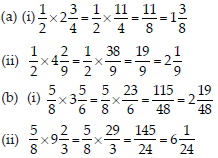Q 16.

Vidya and Pratap went for a picnic. Their mother gave them a water bottle that contained 5 litres of water. Vidya consumed 2/5 of the water. Pratap consumed the remaining water.
(i) How much water did Vidya drink?
(ii) What fraction of the total quantity of water did Pratap drink?

SOLUTION: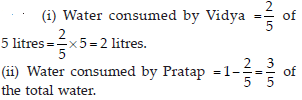Q 17.

Find :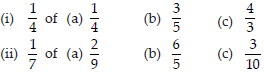SOLUTION: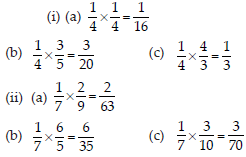Q 18.

Multiply and reduce to lowest form (if possible) :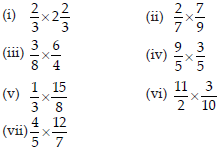SOLUTION: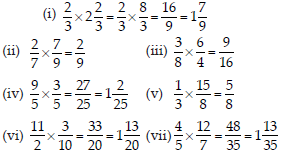Q 19.

Multiply the following fractions: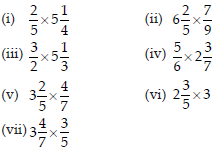SOLUTION: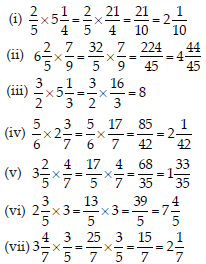Q 20.

Which is greater :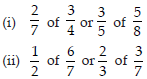SOLUTION: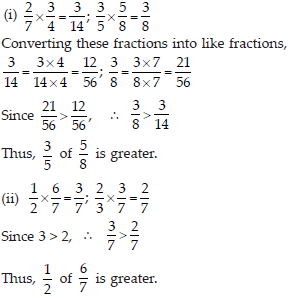Q 21.

Saili plants 4 saplings, in a row, in her garden. The distance between two adjacent saplings is 3/4m. Find the distance between the first and the last sapling.

SOLUTION: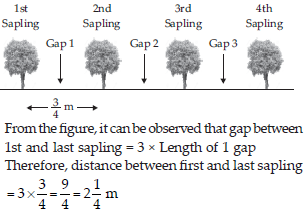Q 22.

Lipika reads a book for 134hours everyday. She reads the entire book in 6 days. How many hours in all were required by her to read the book?

SOLUTION: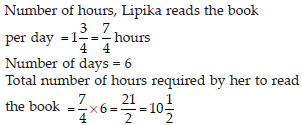Q 23.

A car runs 16 km using 1 litre of petrol. How much distance will it cover using 234 litres of petrol?

SOLUTION: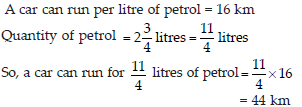Q 24.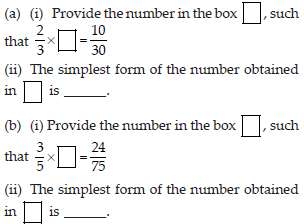SOLUTION: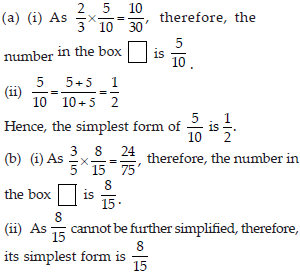Q 25.

Find: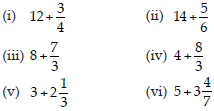SOLUTION: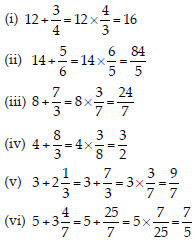Q 26.

Find the reciprocal of each of the following fractions. Classify the reciprocals as proper fractions, improper fractions and whole numbers.
(i) 37 (ii) 58 (iii) 97 (iv) 65 (v) 127 (vi) 18 (vii) 111

SOLUTION: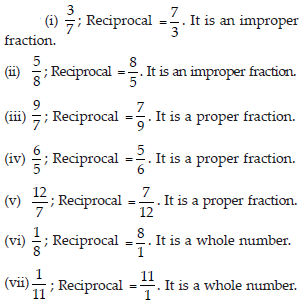Q 27.

Find: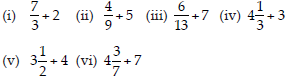SOLUTION: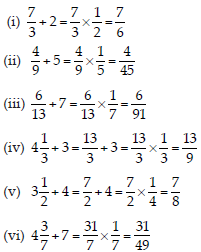Q 28.

Find: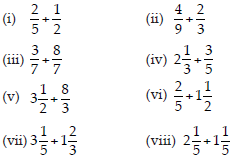SOLUTION: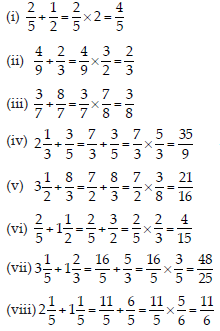Q 29.

Which is greater?
(i) 0.5 or 0.05
(ii) 0.7 or 0.5
(iii) 7 or 0.7
(iv) 1.37 or 1.49
(v) 2.03 or 2.30
(vi) 0.8 or 0.88.

SOLUTION: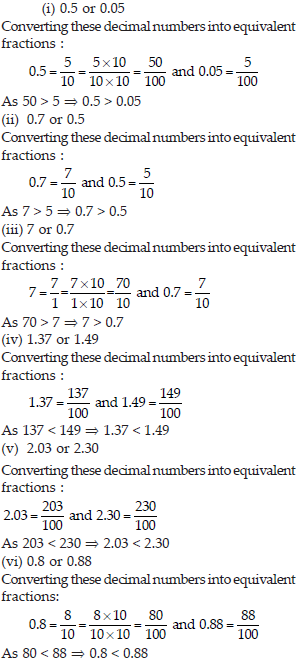Q 30.

Express as rupees using decimals :
(i) 7 paise
(ii) 7 rupees 7 paise
(iii) 77 rupees 77 paise
(iv) 50 paise
(v) 235 paise.

SOLUTION: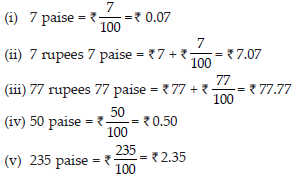Q 31.

(i) Express 5 cm in metre and kilometre.
(ii) Express 35 mm in cm, m and km.

SOLUTION: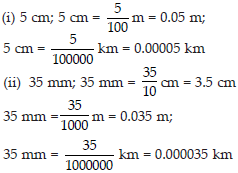Q 32.

Express in kg:
(i) 200 g
(ii) 3470 g
(iii) 4 kg 8 g

SOLUTION: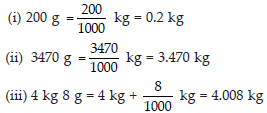Q 33.

Write the following decimal numbers in the expanded form:
(i) 20.03
(ii) 2.03
(iii) 200.03
(iv) 2.034

SOLUTION: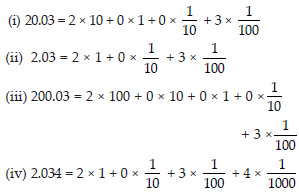Q 34.

Write the place value of 2 in the following decimal numbers:
(i) 2.56
(ii) 21.37
(iii) 10.25
(iv) 9.42
(v) 63.352.

SOLUTION:

(i) 2.56; Ones
(ii) 21.37; Tens
(iii) 10.25; Tenth
(iv) 9.42; Hundredth
(v) 63.352; Thousandth

Q 35.

Dinesh went from place A to place B and from there to place C. A is 7.5 km from B and B is 12.7 km from C. Ayub went from place A to place D and from there to place C. D is 9.3 km from A and C is 11.8 km from D. Who travelled more and by how much?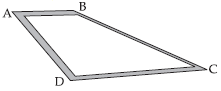SOLUTION:

Distance travelled by Dinesh = AB + BC = (7.5 + 12.7) km = 20.2 km.
Distance travelled by Ayub = AD + DC = (9.3 + 11.8) km = 21.1 km.
Difference = (21.1 – 20.2) km = 0.9 km
Hence, Ayub travelled 0.9 km more than Dinesh.

Q 36.

Shyama bought 5 kg 300 g apples and 3 kg 250 g mangoes. Sarala bought 4 kg 800 g oranges and 4 kg 150 g bananas. Who bought more fruits?

SOLUTION: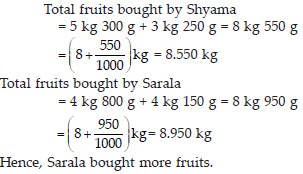Q 37.

How much less is 28 km than 42.6 km?

SOLUTION:

42.6 – 28.0 = 14.6 km
Hence, 28 km is 14.6 km less than 42.6 km.

Q 38.

Find:
(i) 0.2 × 6
(ii) 8 × 4.6
(iii) 2.71 × 5
(iv) 20.1 × 4
(v) 0.05 × 7
(vi) 211.02 × 4
(vii) 2 × 0.86

SOLUTION: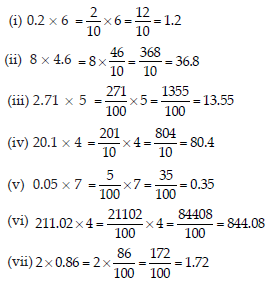Q 39.

Find the area of rectangle whose length is 5.7cm and breadth is 3 cm.

SOLUTION:

Length = 5.7 cm and Breadth = 3 cm
Area = Length × Breadth = 5.7 × 3 = 17.1 cm2

Q 40.

Find:
(i) 1.3 × 10
(ii) 36.8 × 10
(iii) 153.7 × 10
(iv) 168.07 × 10
(v) 31.1 × 100
(vi) 156.1 × 100
(vii) 3.62 × 100
(viii) 43.07 × 100
(ix) 0.5 × 10
(x) 0.08 × 10
(xi) 0.9 × 100
(xii) 0.03 × 1000

SOLUTION:

We know that when a decimal number is multiplied by 10, 100, 1000, the decimal point in the product is shifted to the right by as many places as there are zeroes. Therefore, these products can be calculated as
(i) 1.3 × 10 = 13
(ii) 36.8 × 10 = 368
(iii) 153.7 × 10 = 1537
(iv) 168.07 × 10 = 1680.7
(v) 31.1 × 100 = 3110
(vi) 156.1 × 100 = 15610
(vii) 3.62 × 100 = 362
(viii) 43.07 × 100 = 4307
(ix) 0.5 × 10 = 5
(x) 0.08 × 10 = 0.8
(xi) 0.9 × 100 = 90
(xii) 0.03 × 1000 = 30

Q 41.

A two-wheeler covers a distance of 55.3 km in one litre of petrol. How much distance will it cover in 10 litres of petrol?

SOLUTION:

Distance covered in 1 litre of petrol = 55.3 km
Distance covered in 10 litres of petrol = 10 × 55.3
= 553 km

Q 42.

Find:
(i) 2.5 × 0.3
(ii) 0.1 × 51.7
(iii) 0.2 × 316.8
(iv) 1.3 × 3.1
(v) 0.5 × 0.05
(vi) 11.2 × 0.15
(vii) 1.07 × 0.02
(viii) 10.05 × 1.05
(ix) 101.01 × 0.01
(x) 100.01 × 1.1

SOLUTION: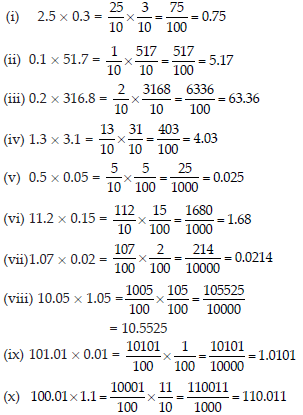Q 43.

Find:
(i) 0.4 ÷ 2
(ii) 0.35 ÷ 5
(iii) 2.48 ÷ 4
(iv) 65.4 ÷ 6
(v) 651.2 ÷ 4
(vi) 14.49 ÷ 7
(vii) 3.96 ÷ 4
(viii) 0.80 ÷ 5

SOLUTION: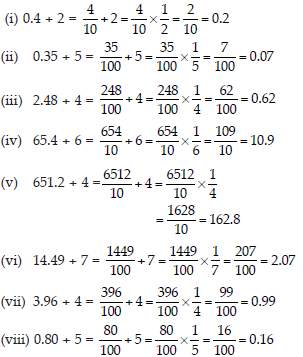Q 44.

Find:
(i) 4.8 ÷ 10
(ii) 52.5 ÷ 10
(iii) 0.7 ÷ 10
(iv) 33.1 ÷ 10
(v) 272.23 ÷ 10
(vi) 0.56 ÷ 10
(vii) 3.97 ÷10

SOLUTION:

We know that when a decimal number is divided by a multiple of 10 (i.e., 10, 100, 1000, etc.), the decimal point will be shifted to the left by as many places as there are zeroes.
(i) 4.8 ÷ 10 = 0.48
(ii) 52.5 ÷ 10 = 5.25
(iii) 0.7 ÷ 10 = 0.07
(iv) 33.1 ÷ 10 = 3.31
(v) 272.23 ÷ 10 = 27.223
(vi) 0.56 ÷ 10 = 0.056
(vii) 3.97 ÷10 = 0.397

Q 45.

Find:
(i) 2.7 ÷ 100
(ii) 0.3 ÷ 100
(iii) 0.78 ÷ 100
(iv) 432.6 ÷ 100
(v) 23.6 ÷100
(vi) 98.53 ÷ 100

SOLUTION:

We know that when a decimal number is divided by a multiple of 10 (i.e., 10, 100, 1000, etc.), the decimal point will be shifted to the left by as many places as there are zeroes.
(i) 2.7 ÷ 100 = 0.027
(ii) 0.3 ÷ 100 = 0.003
(iii) 0.78 ÷ 100 = 0.0078
(iv) 432.6 ÷ 100 = 4.326
(v) 23.6 ÷100 = 0.236
(vi) 98.53 ÷ 100 = 0.9853

Q 46.

Find:
(i) 7.9 ÷ 1000
(ii) 26.3 ÷ 1000
(iii) 38.53 ÷ 1000
(iv) 128.9 ÷ 1000
(v) 0.5 ÷ 1000

SOLUTION:

We know that when a decimal number is divided by a multiple of 10 (i.e., 10, 100, 1000, etc.), the decimal point will be shifted to the left by as many places as there are zeroes.
(i) 7.9 ÷ 1000 = 0.0079
(ii) 26.3 ÷ 1000 = 0.0263
(iii) 38.53 ÷ 1000 = 0.03853
(iv) 128.9 ÷ 1000 = 0.1289
(v) 0.5 ÷ 1000 = 0.0005

Q 47.

Find:
(i) 7 ÷ 3.5
(ii) 36 ÷ 0.2
(iii) 3.25 ÷ 0.5
(iv) 30.94 ÷ 0.7
(v) 0.5 ÷ 0.25
(vi) 7.75 ÷ 0.25
(vii) 76.5 ÷ 0.15
(viii)37.8 ÷ 1.4
(ix) 2.73 ÷ 1.3

SOLUTION: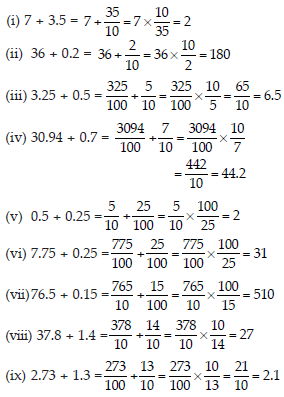Q 48.

A vehicle covers a distance of 43.2 km in 2.4 litres of petrol. How much distance will it cover in one litre of petrol?

SOLUTION: